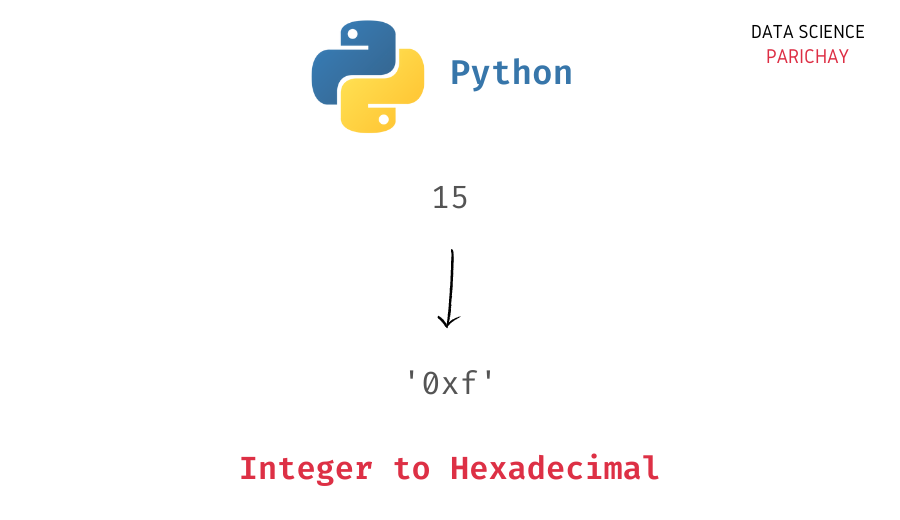# Python – Convert Integer to Hexadecimal

In this tutorial, we will look at how to convert an integer to hexadecimal in Python with the help of some examples.

## How to convert integer to hexadecimal in Python?

You can use the Python built-in `hex()` function to convert an integer to its hexadecimal form in Python. Pass the integer as an argument to the function. The following is the syntax –

```# convert int i to hexadecimal
hex(i)```

It returns the integer’s representation in the hexadecimal number system (base 16) as a lowercase string prefixed with `'0x'`.

## Examples

Let’s look at some examples of using the above function to convert int to hex.

Pass the integer as an argument to hex function.

```# int variable
num = 15
# int to hex
num_hex = hex(num)
# display hex and type
print(num_hex)
print(type(num_hex))```

Output:

```0xf
<class 'str'>```

You can see that we get the hexadecimal representing the integer as a lowercase string with `'0x'` prefix.

If you do not want the prefix, you can use the string slice operation to remove the prefix from the returned hex string.

📚 Data Science Programs By Skill Level

Introductory

Intermediate ⭐⭐⭐

🔎 Find Data Science Programs 👨‍💻 111,889 already enrolled

Disclaimer: Data Science Parichay is reader supported. When you purchase a course through a link on this site, we may earn a small commission at no additional cost to you. Earned commissions help support this website and its team of writers.

Let’s now apply the same function to a negative integer.

```# int variable
num = -15
# int to hex
num_hex = hex(num)
# display hex and type
print(num_hex)
print(type(num_hex))```

Output:

```-0xf
<class 'str'>```

### Integer to uppercase hexadecimal string

Alternatively, you can use the Python built-in `format()` function to convert an integer to its hexadecimal form. You can customize the format of the returned string.

For example, to convert an integer to an uppercase hexadecimal string, use the format string `'#X'` or `'X'`.

```# int variable
num = 15
# int to hex
num_hex = format(num, '#X')
# display hex and type
print(num_hex)
print(type(num_hex))```

Output:

```0XF
<class 'str'>```

We get the hexadecimal for the integer as an uppercase string.

With the `format()` function you can customize the returned hexadecimal string – with or without the prefix, uppercase or lowercase, etc.

```# int variable
num = 15
# int to hex
print(f"Lowercase with prefix: {format(num, '#x')}")
print(f"Lowercase without prefix: {format(num, 'x')}")
print(f"Uppercase with prefix: {format(num, '#X')}")
print(f"Uppercase without prefix: {format(num, 'X')}")```

Output:

```Lowercase with prefix: 0xf
Lowercase without prefix: f
Uppercase with prefix: 0XF
Uppercase without prefix: F```

You might also be interested in –

•